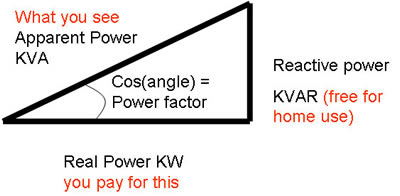# Difference between kVa and kW

##### Key difference: kVa and kW are power factors. kVa is a unit of apparent power, which comprises of real and re-active powers, whereas kW is a unit of only real power.

The electrical appliances and machinery expresses the units in kVa and kW, these are electrical units and ratings generally used to indicate the power requirement and consumptions. The unit kVa and kW are different in AC (Alternate Current) and DC (Direct Current); that is in AC the kVa need to be converted, whereas in DC it need not be converted.

kVa is a product of kV (kilo-volts) and amperes. It values is 1000 amperes. Basically, it is known as ‘apparent power’ of a specific circuit and appliances in an electrical system. It is product of apparent and real power factors. A term generally refers to the apparent power that is the absolute value of complex power, (S) and is equal to the product of the volts and amps. The amount of kVa actually utilized for doing work is less, and the rest of the amount includes the excess current.

kW is commonly known as ‘real power’, for a particular circuits in the electrical systems. It is simply the amount of actual power that does valid work. The kW solving requires an additional variable called as the Power factor (PF), which keeps on fluctuating according to the different electrical appliances. One kW is equal to 1000 watts. An appliance or machinery utilizes less amount of kW, when the voltage is out of phase with that of the current.The associative formulas and conversions used in kVa and kW are:

• VA = Voltage x Amps
• Watts = Voltage (root-mean-squared) x Amps (root-mean-squared) x Power Factor (PF) (a three-phase circuit would multiply the voltage by the square root of 3 or approximately 1.732).

The relationship between the three (kVA, kW, and Power Factor) is mathematically described as:

• kW = kVA x Power Factor;
• kVA = kW / Power Factor;
• Power Factor = kW / kVA

In DC circuits, the power factor is mathematically inconsequential, because it is in unity. Hence it is expressed as:

• kW = kVA = Volts x Current x 1 = Volts x Current

Comparison between kVa and kW:

 kVa kW They are kVa is the unit of apparent power, which includes real and re-active power. kW is the unit of only real power. Stands for kVa is kilo Volt amperes kW is kiloWatts Refer to kVa is refers to the only portion available to do work. kW is the amount of power capable of doing work. Conversion 1 kVa= 0.8 kW 1 kW=1.25 kVa Term applicable in countries kVa is usually used when referring to the generators in majority of the countries other than US in the world. KW is usually used when referring to the generators in the United States.

Image Courtesy: nlcpr.com